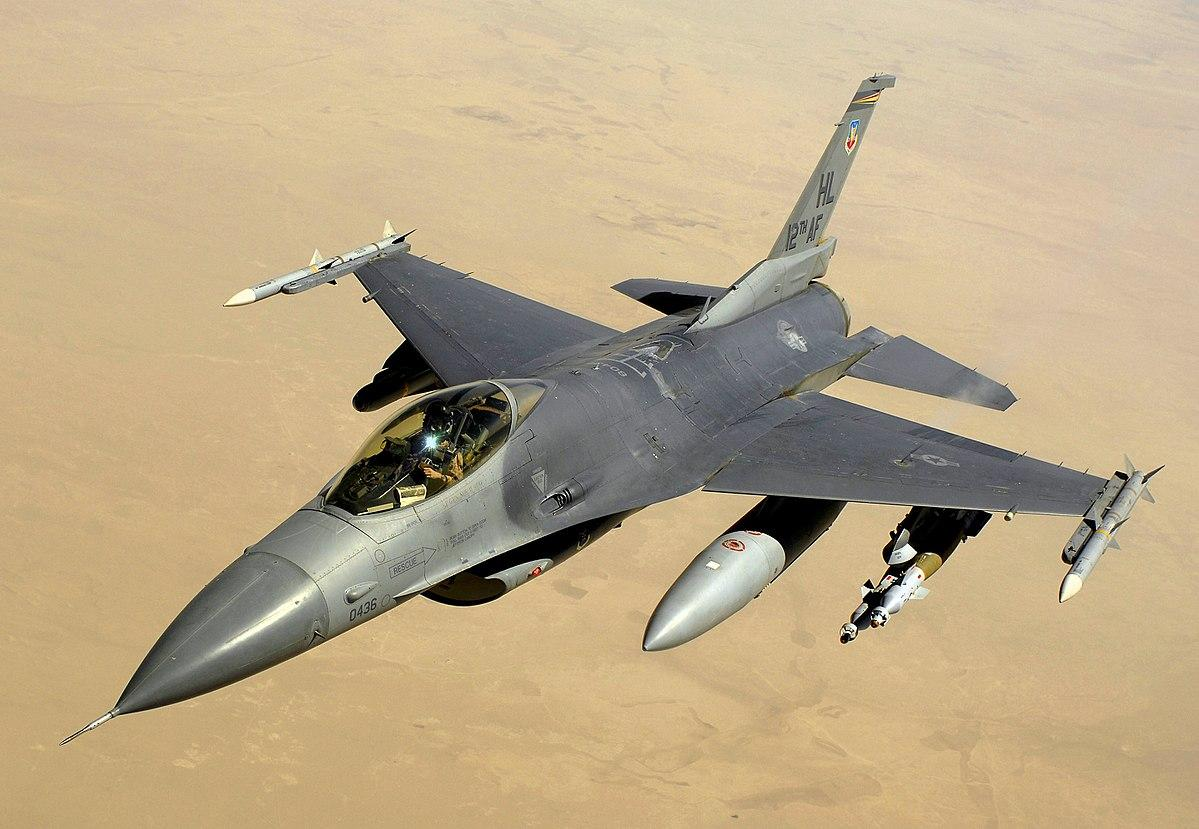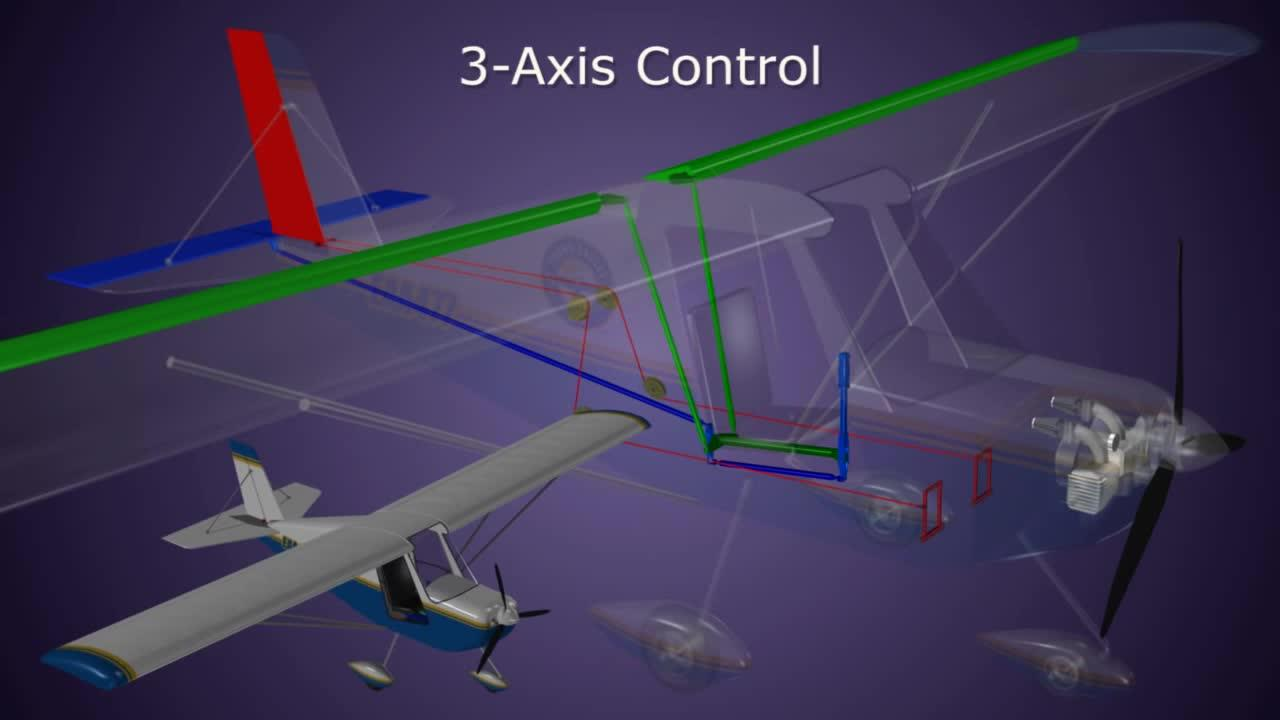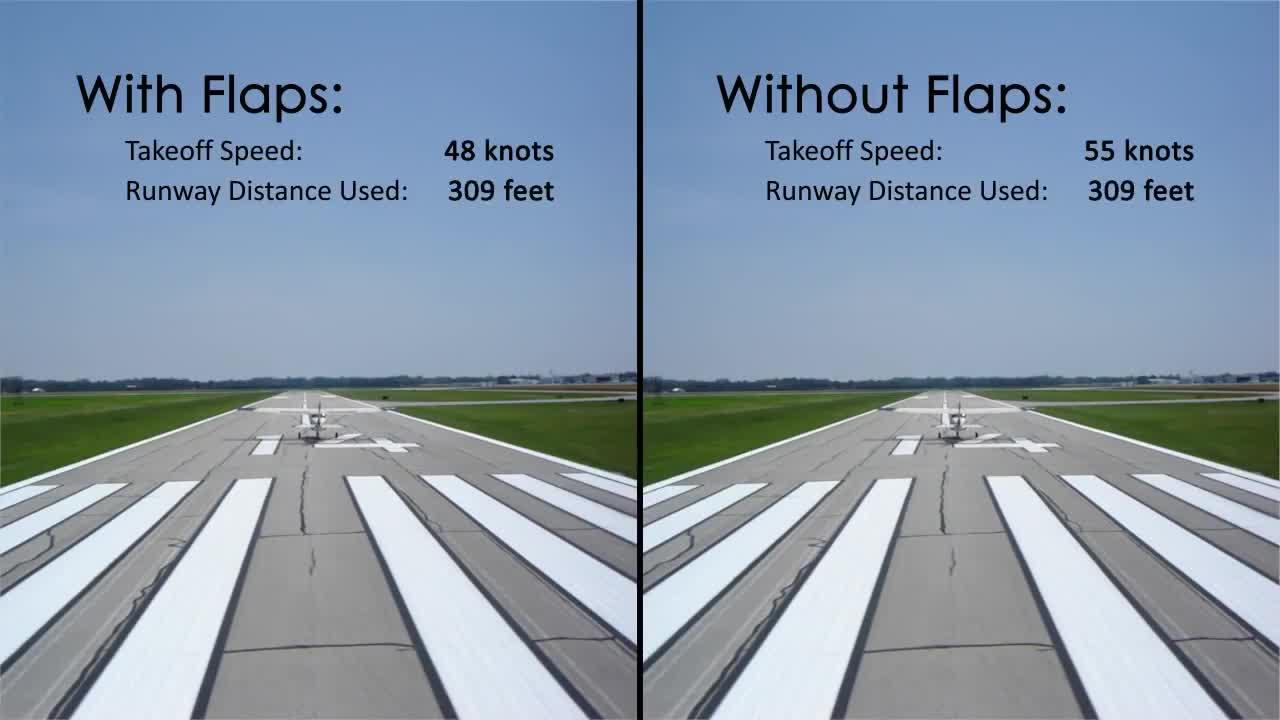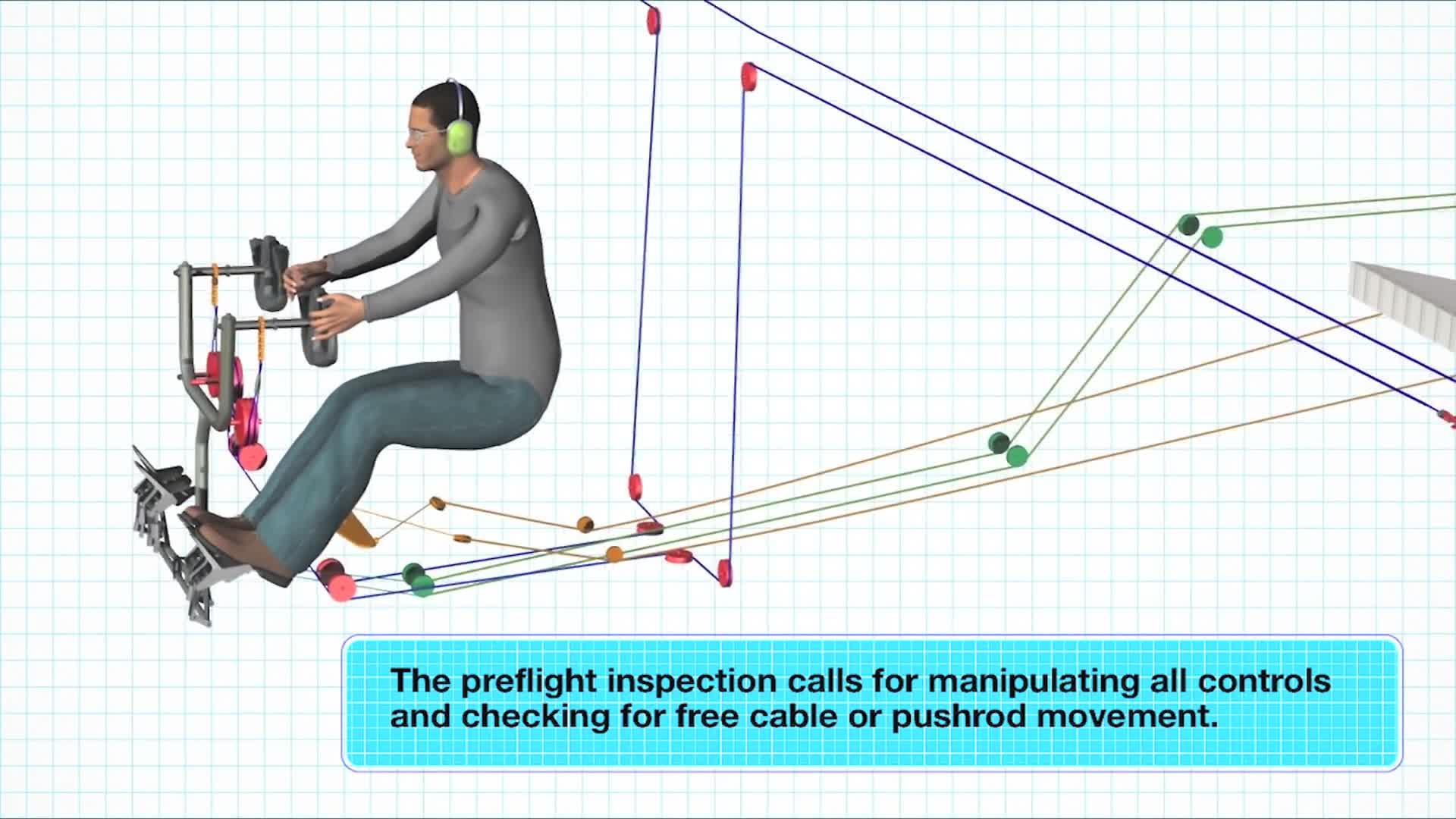一、飞行控制系统的发展历程
1.1 机械操纵系统
1.2 增稳系统
1.3 控制增稳系统

3.1 内环为迎角反馈
3.2 内环为角速率反馈
3.3 内环为迎角+角速率反馈

4.1 以过载作为控制指令
4.2 以C*作为控制指令
4.3 加入作动器和滤波器模型F16飞机https://www.zhihu.com/video/1177699837007663104

1903莱特兄弟第一次有动力的飞行表明，飞机除了自身的稳定性外，驾驶员对于飞机操纵的可控性，同样具有重要意义。可以说，飞行控制技术是与飞机设计技术同步发展的，下面我们就来看一看飞行控制技术是如何演变的。

## 一、飞行控制系统的发展历程

### 1.1 机械操纵系统Youtube, author: Kevin Thorphttps://www.zhihu.com/video/1177370296414412800author: ERAUSpecialVFRhttps://www.zhihu.com/video/1177370644215365632Youtube, author:shidifu111https://www.zhihu.com/video/1177370710182494208

## 二、简化的纵向短周期状态方程true velocity (350-900 ft/sec):800
altitude(0-40000ft ft):30000
center of gravity position (0.2-0.5, reference cxg=0.35) 0.5%%
U=800;g=32.15;
Za=-574.8800;Zq=-28.4000;Ma=13.9842;Mq=-0.0943;
Zde=-1.0400;Mde=-0.1476;

A=[Za/U 1+Zq/U;Ma Mde];B=[Zde/U;Mde];C=[1 0;0 1];D=0;
damp(ss(A,B,C,D))

    Pole        Damping       Frequency      Time Constant
3.25e+00    -1.00e+00       3.25e+00        -3.08e-01
-4.12e+00     1.00e+00       4.12e+00         2.43e-01 

## 三、SAS（增稳系统）系统设计

### 3.1 内环为迎角反馈%%
clc
U=800;g=32.15;
Za=-574.8800;Zq=-28.4000;Ma=13.9842;Mq=-0.0943;
Zde=-1.0400;Mde=-0.1476;
A=[Za/U 1+Zq/U;Ma Mde];B=[Zde/U;Mde];C=[-1 0];D=0;

sys=ss(A,B,C,D)；h=rlocusplot(sys);sgrid
p=getoptions(h);
p.Xlabel.FontSize=12;p.Ylabel.FontSize=12;
p.TickLabel.FontWeight='demi';p.TickLabel.FontSize=10;
setoptions(h,p);
set(findobj('Type','line'),'linewidth',2,'MarkerSize',10)

         Pole              Damping       Frequency      Time Constant
-4.98e-01 + 7.91e-01i     5.33e-01       9.35e-01         2.01e+00
-4.98e-01 - 7.91e-01i     5.33e-01       9.35e-01         2.01e+00   

U=800;g=32.15;
Za=-574.8800;Zq=-28.4000;Ma=13.9842;Mq=-0.0943;
Zde=-1.0400;Mde=-0.1476;

A=[Za/U 1+Zq/U;Ma Mde];B=[Zde/U;Mde];C=[1 0;0 1];D=0;
K=[-100 0];
damp(ss(A-B*K,B,C,D))

### 3.2 内环为角速率反馈• 以B747为例

B747在某飞行状态下纵向短周期方程为：Pole              Damping       Frequency      Time Constant
-4.50e-01 + 7.20e-01i     5.31e-01       8.49e-01         2.22e+00
-4.50e-01 - 7.20e-01i     5.31e-01       8.49e-01         2.22e+00  

• 以F16为例

### 3.3 内环为迎角+角速率反馈%%
clc
U=800;g=32.15;
Za=-574.8800;Zq=-28.4000;Ma=13.9842;Mq=-0.0943;
Zde=-1.0400;Mde=-0.1476;
A=[Za/U 1+Zq/U;Ma Mde];B=[Zde/U;Mde];C=[0 -1];D=0;

p1=-1.6+1.2i;
p2=-1.6-1.2i;
K=place(A,B,[p1 p2]);

 damp(ss(A-B*K,B,C,D))

         Pole              Damping       Frequency      Time Constant
-1.60e+00 + 1.20e+00i     8.00e-01       2.00e+00         6.25e-01
-1.60e+00 - 1.20e+00i     8.00e-01       2.00e+00         6.25e-01   

## 四、CAS（控制增稳系统）系统设计

### 4.1 以过载作为控制指令以及%%
U=800;g=32.15;
Za=-574.8800;Zq=-28.4000;Ma=13.9842;Mq=-0.0943;
Zde=-1.0400;Mde=-0.1476;

A=[Za/U 1+Zq/U;Ma Mq];
B=[Zde/U;Mde];
C=[-Za/g -Zq/g];
D=-Zde/g;

p1=-20;
p2=-1.6+1.2i;
p3=-1.6-1.2i;
Aa=[A [0;0];C 0]
Ba=[B;D]
K=place(Aa,Ba,[p1 p2 p3])

K = -414.2893 -153.6963  -25.8763### 4.2 以C*作为控制指令

C*可定义成：U=800;g=32.15;
Za=-574.8800;Zq=-28.4000;Ma=13.9842;Mq=-0.0943;
Zde=-1.0400;Mde=-0.1476;

A=[Za/U 1+Zq/U;Ma Mq];
B=[Zde/U;Mde];
C=[-Za/g -Zq/g+12.4];
D=-Zde/g;

p1=-20;
p2=-1.6+1.2i;
p3=-1.6-1.2i;
Aa=[A [0;0];C 0]
Ba=[B;D]
K=place(Aa,Ba,[p1 p2 p3])

K= -200.1392 -153.6693  -17.2702

C*指令的响应曲线如下，可见可以很好的实现很好的跟随。

### 4.3 加入作动器和滤波器模型%%
U=800;g=32.15;
Za=-574.8800;Zq=-28.4000;Ma=13.9842;Mq=-0.0943;
Zde=-1.0400;Mde=-0.1476;

A=[Za/U 1+Zq/U;Ma Mq];
B=[Zde/U;Mde];
C=[-Za/g -Zq/g+12.4];
D=-Zde/g;

p1=-20;
p2=-1.6+1.2i;
p3=-1.6-1.2i;
A=[A [0;0];C 0]
B=[B;D]
K=place(A,-B,[p1 p2 p3])%注意此时作动器简化为-1，因此B前面增加了负号

K =  200.1392  153.6963   17.2702

%%
clc;clear
U=800;g=32.15;
Za=-574.8800;Zq=-28.4000;Ma=13.9842;Mq=-0.0943;
Zde=-1.0400;Mde=-0.1476;

A=[Za/U 1+Zq/U;Ma Mq];
B=[Zde/U;Mde];
C=[-Za/g -Zq/g+12.4];
D=-Zde/g;

H=[-Za/g -Zq/g+12.4 -Zde/g 0 0];

Aa=[A B [0 0;0 0];[0 0 -20 0 0];[10 0 0 -10 0];H];
Ba=[0 0 -20 0 0]';

Ca=[0 0 0 1 0;0 1 0 0 0;H;0 0 0 0 1];
Ga=[0 0 0 0 -1]';
Da=[0 0 -1 0]';

p1=-20;
p2=-1.6+1.2i;
p3=-1.6-1.2i;
A=[A [0;0];C 0];
B=[B;D];
K=place(A,-B,[p1 p2 p3]);
K=[K(1) K(2) 0 K(3)];
damp(ss(Aa-Ba*K*Ca,Ga-Ba*K*Da,Ca,Da))
step(ss(Aa-Ba*K*Ca,Ga-Ba*K*Da,Ca,Da),10)

         Pole              Damping       Frequency      Time Constant
-2.13e+00 + 1.02e+00i     9.01e-01       2.36e+00         4.70e-01
-2.13e+00 - 1.02e+00i     9.01e-01       2.36e+00         4.70e-01
-7.27e+00                 1.00e+00       7.27e+00         1.37e-01
-9.64e+00 + 1.74e+01i     4.85e-01       1.99e+01         1.04e-01
-9.64e+00 - 1.74e+01i     4.85e-01       1.99e+01         1.04e-01 

%%
h=rlocusplot(ss(Aa-Ba*K*Ca,Ba,H,0));
sgrid
p=getoptions(h);
p.Xlabel.FontSize=12;
p.Ylabel.FontSize=12;
p.Xlim=[-12 1];
p.Ylim=[-50 50];
p.TickLabel.FontWeight='demi';
p.TickLabel.FontSize=10;
setoptions(h,p);
set(findobj('Type','line'),'linewidth',2,'MarkerSize',10)